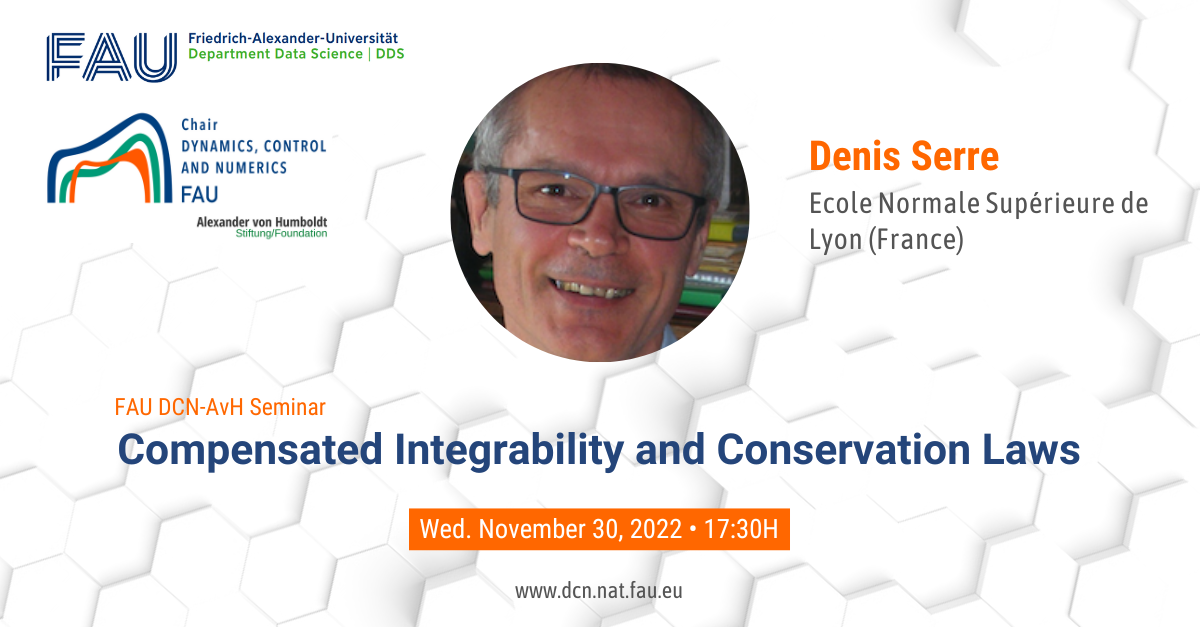# Compensated Integrability and Conservation LawsDate: Wed. November 30, 2022
Event: FAU DCN-AvH Seminar
Organized by: FAU DCN-AvH, Chair for Dynamics, Control and Numerics – Alexander von Humboldt Professorship at FAU Friedrich-Alexander-Universität Erlangen-Nürnberg (Germany)
Title: FAU DCN-AvH Seminar “Compensated Integrability and Conservation Laws”

Speaker: Prof. Dr. Denis Serre
Affiliation: Ecole Normale Supérieure de Lyon (France).

Abstract. Compensated Integrability is a recent tool from Functional Analysis. It applies to positive semi-definite tensors whose row-wise Divergence is a finite measure. Quite often, this Divergence vanishes identically. We shall explain why Div-free tensors occur naturally in various models of Mathematical Physics, as a consequence of N{\oe}ther’s Theorem.

Somehow, Compensated Integrability is dual to Brenier’s existence result for the “second BVP” for the Monge-Ampère equation. It extends in a non-trivial manner classical statements, such as Gagliardo-Nirenberg-Sobolev Inequality, or the Isoperimetric Inequality. In the periodic situation, it expresses the Div-quasiconcavity of A\mapsto(\det A)^{\frac1{n-1}} (recall that A\mapsto(\det A)^{\frac1n} is concave over {\bf Sym}_n^+). This leads to a weak upper-semicontinuity result (collaboration with L. De Rosa & R. Tione).

When it applies, C.I. yields dispersive (Strichartz-like) estimates. We thus learn that in Gas Dynamics, the internal energy cannot concentrate on zero-measure subsets. Other applications concern various models for particle dynamics: kinetic equations (Boltzman), mean-field models (Vlasov), molecular dynamics. The corresponding tensor is positive semi-definite whenever the particles interact pairwise according to a radial, repulsive force. Hard spheres dynamics shows that a Div-free tensor can be supported by a small set (here a graph), in which case a special form of C.I. is required.

Another relevant topic is that of multi-dimensional conservation laws, where it allows us to extend Kruzkov’s theory to L^p-data when p is finite, under a non-degeneracy assumption (collaboration with L. Silvestre).

Slides